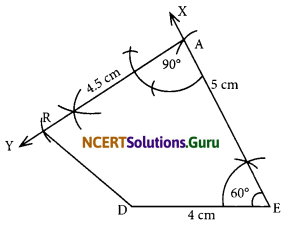# NCERT Solutions for Class 8 Maths Chapter 4 Practical Geometry Ex 4.4

These NCERT Solutions for Class 8 Maths Chapter 4 Practical Geometry Ex 4.4 Questions and Answers are prepared by our highly skilled subject experts.

## NCERT Solutions for Class 8 Maths Chapter 4 Practical Geometry Exercise 4.4

Question 1.
DE = 4 cm
EA = 5 cm
AR = 4.5 cm
∠E = 60°
∠A = 90°TR = 3.5 cm
RU = 3 cm
UE = 4 cm
∠R = 75°
∠U = 120°
Solution:
(i)Steps of construction:
I. Draw a line segment DE = 4 cm.
II. At E, draw $$\overrightarrow{\mathrm{EX}}$$ such that ∠DEX = 60°.
III. From $$\overrightarrow{\mathrm{EX}}$$, cut-off EA = 5 cm.
IV. At A, draw ray $$\overrightarrow{\mathrm{AY}}$$ such that ∠EAY = 90°.
V. From $$\overrightarrow{\mathrm{AY}}$$, cut-off AR = 4.5 cm.
VI. Join R and D. Thus, DEAR is the required quadrilateral.(ii)Steps of construction:
I. Draw a line segment $$\overrightarrow{\mathrm{TR}}$$ = 3.5 cm.
II. At R, draw a ray $$\overrightarrow{\mathrm{RX}}$$, such that ∠TRX = 75°
III. From $$\overrightarrow{\mathrm{RX}}$$, cut-off RU = 3 cm.
IV. At U, draw $$\overrightarrow{\mathrm{UY}}$$ such that ∠RUY = 120°.
V. From UY, cut-off $$\overrightarrow{\mathrm{UE}}$$ = 4 cm.
VI. Join E and T. Thus, TRUE is the required quadrilateral.

error: Content is protected !!